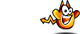Unit Conversion Software Web Widgets Loan Calculator Currency Rates Country Flags Unit Converter
 Faq Help# Energy and Work ConverterContact
 From: To: attojoule [aJ]Btu (IT) [Btu (IT), Btu]Btu (th) [Btu (th)]calorie (IT) [cal (IT), cal]calorie (nutritional) [cal (nutritional)]calorie (th) [cal (th)]dyne centimeter [dyn*cm]electron-volt [eV]ergfoot-pound [ft*lbf]fuel oil equivalent @barrel (US)fuel oil equivalent @kilolitergigajoule [GJ]gigaton [Gton]gigawatt-hour [GW*h]gram-force centimeter [gf*cm]gram-force meter [gf*m]Hartree energyhorsepower (metric) hourhorsepower hour [hp*h]inch-ounce [in*ozf]inch-pound [in*lbf]joule [J]kilocalorie (IT) [kcal (IT), kcal]kilocalorie (th) [kcal (th)]kiloelectron-volt [keV]kilogram-force centimeter [kgf*cm]kilogram-force meter [kgf*m]kilojoule [kJ]kilopond meter [kp*m]kiloton [kton]kilowatt-hour [kW*h]kilowatt-second [kW*s]mega Btu (IT) [MBtu (IT), MBtu]megaelectron-volt [MeV]megajoule [MJ]megaton [Mton]megawatt-hour [MW*h]microjoule [µJ]millijoule [mJ]nanojoule [nJ]newton meter [N*m]ounce-force inch [ozf*in]pound-force foot [lbf*ft]pound-force inch [lbf*in]poundal foot [pdl*ft]Rydberg constantthermtherm (EC)therm (US)ton (explosives)ton-hour (refrigeration) [ton*h]watt-hour [W*h]watt-second [W*s] attojoule [aJ]Btu (IT) [Btu (IT), Btu]Btu (th) [Btu (th)]calorie (IT) [cal (IT), cal]calorie (nutritional) [cal (nutritional)]calorie (th) [cal (th)]dyne centimeter [dyn*cm]electron-volt [eV]ergfoot-pound [ft*lbf]fuel oil equivalent @barrel (US)fuel oil equivalent @kilolitergigajoule [GJ]gigaton [Gton]gigawatt-hour [GW*h]gram-force centimeter [gf*cm]gram-force meter [gf*m]Hartree energyhorsepower (metric) hourhorsepower hour [hp*h]inch-ounce [in*ozf]inch-pound [in*lbf]joule [J]kilocalorie (IT) [kcal (IT), kcal]kilocalorie (th) [kcal (th)]kiloelectron-volt [keV]kilogram-force centimeter [kgf*cm]kilogram-force meter [kgf*m]kilojoule [kJ]kilopond meter [kp*m]kiloton [kton]kilowatt-hour [kW*h]kilowatt-second [kW*s]mega Btu (IT) [MBtu (IT), MBtu]megaelectron-volt [MeV]megajoule [MJ]megaton [Mton]megawatt-hour [MW*h]microjoule [µJ]millijoule [mJ]nanojoule [nJ]newton meter [N*m]ounce-force inch [ozf*in]pound-force foot [lbf*ft]pound-force inch [lbf*in]poundal foot [pdl*ft]Rydberg constantthermtherm (EC)therm (US)ton (explosives)ton-hour (refrigeration) [ton*h]watt-hour [W*h]watt-second [W*s] Result:How to use Energy and Work Converter
Select the unit to convert from in the input units list. Select the unit to convert to in the output units list. Enter the value to convert from into the input box on the left. The conversion result will immediately appear in the output box.Download Energy and Work Unit Converter
our powerful software utility that helps you make easy conversion between more than 2,100 various units of measure in more than 70 categories. Discover a universal assistant for all of your unit conversion needs - download the free demo version right away!Instantly add a free Energy and Work Converter Widget to your website
It will take less than a minute, is as easy as cutting and pasting. The converter will blend seamlessly into your website since it is fully rebrandable.Looking for an interactive energy and work conversion table? Visit our forum to discuss conversion issues and ask for free help! Try the instant categories & units searchit gives you results as you type!Copyright © 1998-2009 UnitConversion.org Privacy & Terms | About | Faq | Help | Contact | Link to Us